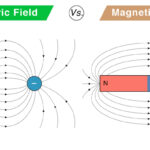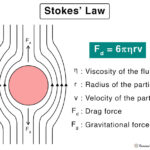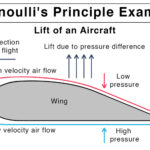Home / Physics / Static Friction

# Static Friction

## What is Static Friction

Suppose an object lies motionless on a surface. A force is applied in order to displace it. However, the object continues to remain stationary. The reason is that the surface exerts a reaction force in a direction opposite to the applied force. This force is known as the frictional force. Because the object remains immobile, the phenomenon of resisting its motion is known as static friction.

The static friction force always occurs at the contact region between the object and the surface. Essentially, it takes place between two surfaces in contact. When the applied force is large enough to move the object, the static friction disappears, and kinetic friction comes into action.

## Static Friction Examples

Static friction is responsible for keeping an object at rest. Here are some examples.

• A book lying on a table
• A car parked on a hill
• A towel hanging from a rack
• A person walking on a floor

## Characteristics of Static Friction

Here are some facts and properties of static friction.

• Contact force
• Responsive force
• Reaches a maximum value before kinetic friction takes over

## Laws of Static Friction

Here are some laws that the force of static friction obeys.

• Proportional to the normal force
• Equal and opposite to the applied force
• Dependent on the surface roughness
• Independent on the area of contact between two surfaces

## How to Calculate Static Friction

The static friction can be calculated by applying principles of physics, especially Newton’s laws of motion.

### Static Friction Equation

The static friction equation can be derived from the laws of friction.

1. Horizontal Surface

Suppose an object of mass m is lying motionless on a table. When a force F is applied to it, the table responds by exerting an equal and opposite force at the contact region. This force is known as static friction FS. According to the above laws, FS is proportional to the normal force FN.

FS FN

Or, FS = μS FN

The constant of proportionality is known as the coefficient of static friction. It is a unitless and dimensionless quantity whose value depends upon the nature of surfaces. Smooth surfaces have low values, whereas rough surfaces have high values.

The weight W of the object is given by

W = mg

From Newton’s third law of motion, the weight is balanced by the normal force.

W = FN

Or, FN = mg

Therefore, the formula for the magnitude of static friction is given by

FS = μS mg

Work done W by force is given by the product of force and displacement d.

W = F x d

Since the static friction force does not displace the object, therefore d = 0 and hence,

W = FS x 0 = 0

The work done by static friction is zero.

2. Incline Plane

Suppose the block is motionless on an inclined plane that makes an angle θ with the horizontal.  Its weight mg can be resolved into two components – one parallel to the surface and the other perpendicular to the surface.

Perpendicular component: mg cos θ

From Newton’s third law, the normal force is

FN = mg cos θ

Applying the friction laws as before, the magnitude of static friction is

FS = μS mg cos θ

Therefore, the static friction is dependent on the angle of the ramp. As the angle is increased, the object starts to move, and kinetic friction takes over.

Parallel component: mg sin θ

When the angle of inclination is increased, the block starts to move. Assuming that the block moves with a constant velocity such that there is no acceleration, then mg sin θ must be equal to the frictional force.

mg sin θ = FS

Or, mg sin θ = μS mg cos θ

Or, μS = tan θ

3. Circular Motion

Suppose a car of mass M is moving with a velocity V around in a circle. It will have a force due to its weight W, balanced by the normal load FL.

FL = W = Mg

It will also experience a centripetal force FC directed away from the center of the circle. If R is the radius of the circular path, then the centripetal force is,

FC = MV2/R

Since the car is not skidding, the centripetal force will be balanced by the static friction FS, which is given by

FS = μS FL = μS Mg

And,

FC = FS

Or, MV2/R = μS Mg

Or, μS = V2/gR

The above equation gives the value of the coefficient of static friction needed to provide the desired friction force to allow a car, traveling at speed V, to make it around a flat curve of radius R.

## Limiting Friction

When the applied force is high enough to move the object, then static friction ceases to exist. Just before the object moves, the static friction force reached a maximum value. The limiting friction FL is defined as the maximum value of the static friction.

FL = (FS)max

## How to Reduce Static Friction

The static friction arises due to molecular adhesion between two surfaces. Aside, surfaces consist of tiny bumps that come in contact and inhibit the motion. One way to reduce friction is by polishing the surfaces such that the bumps disappear, making the surfaces smooth. In that way, less force will be required to slide one surface over another. Another way is by introducing a layer of lubricant between surfaces. Lubricants provide a barrier between the two surfaces such that their bumps do not come in contact. Finally, it is also possible to reduce static friction by introducing ball bearings. In this situation, one surface rolls smoothly over another, thus converting static friction into rolling friction.

• Makes motion on a surface possible (e.g., walking, running, driving, skating, and writing)
• Prevents slipping and sliding (e.g., a ladder can stay on the wall, and objects can pile up on top of one another)
• Allows vehicles to stop using brakes

• Makes it hard to push and pull an object
• Makes us slip on ice due to low friction

## Applications of Static Friction

The static friction finds its way in industrial applications.

• Friction welding stabilizes and strengthens cold welded joints

## Difference between Static and Kinetic Friction

The static friction between the two surfaces is higher than the kinetic friction. Static friction is due to the forces of adhesion between surfaces. A large force is required to overcome these adhesive forces. When the applied force is equal to the static friction force, the surfaces will start to move relative to one another. Then, kinetic friction comes into action.Electric Field vs. Magnetic FieldHooke’s LawStokes’ LawBernoulli’s Principle and Equation